10 Apr, 2015

# Estimating Sour Gas Water Content by New Correlations and Simplified Charts

Water is produced with oil and gas. A question that comes to mind is: “Why is water important?” The presence of water may cause corrosion, freezing and hydrate formation. All of these problems are enhanced by the presence of acid gases such as H2S and CO2. The water content of a gas depends on the system temperature, pressure and composition of the water (containing gas). Sour gas will hold more water than sweet gas at the typical operating conditions encountered in a natural gas dehydration facility. There are several methods of calculating the water content of sour gases. The details of these methods can be found in Chapter 6 of Volume 1 of “Gas Conditioning and Processing”  and Chapter 20 of the GPSA (Gas Processors and Suppliers Association) data book .

In the November 2007 Tip of the Month (TOTM), we discussed the phase behavior of water-saturated sour gases. Using limited experimental data, we also demonstrated the accuracy of Maddox et al. shortcut method  and a rigorous calculation method based an equation of state.

In this TOTM, we will present a set of correlations and simplified charts for estimating sour gas water content directly without having to look up the water content of sweet gas. These correlations are based on the Wichert and Wichert chart  (Figure 20-9 of the GPSA data book) and Wagner and Pruss water vapor equation  and Bukacek correlation for estimating sweet gas water content . The proposed correlations are valid for pressures up to 24 MPa (3500 psia), temperatures up to 175°C (350°F) and H2S equivalent concentrations of up to 50 mole %. The accuracy of the proposed correlations was compared against limited experimental data and a rigorous method using an equation of state.

Proposed Correlations and Charts

The vapor pressure of pure water, from 0 to 360, (32 to 680) can be calculated by Wagner-Pruss equation .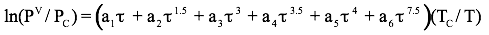(1)

Where:

τ = 1 – (T/TC)

The critical temperature, TC = 647.096 K and critical pressure, PC = 22.064 MPa, T in K, and PV in MPa, and

a1 = −7.85951783,     a2 = 1.84408259,     a3 = −11.7866497,      a4 = 22.6807411,

a5 = −15.9618719,     a6 = 1.80122502

Bukacek  suggested a relatively simple correlation for the water content of lean sweet gas as follows: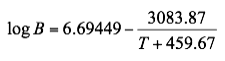(2)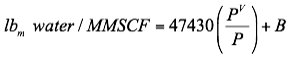(3)

where T is in °F and PV and P should have the same unit (either kPaa or psia). This correlation is reported to be accurate for temperatures between 15.5 and 238°C (60 and 460°F) and for pressure from 0.10 to 70 MPa (15 to 10,000 psia).

Based on the Wichert and Wichert chart, the sour gas water content is estimated by multiplying the sweet gas water content by a sourness correction factor, F. This correction factor, F, is a function of H2S equivalent concentration (HEC), temperature and pressure. The H2S equivalent concentration is defined by:

HEC = Mole% H2S + 0.7 (Mole % CO2

To develop the proposed correlation, we have defined an intermediate term represented by X, as a function of temperature and HEC;, i.e, X = f (T, HEC).

X = α + βT + γT2 (5)

where T is in °F and:

α = 195.262 / [1+26.162 e(-0.0957HEC)] (6)

β = -0.8374 / [1+ 27.813 e(-0.0991HEC)] (7)

γ = 0.0011 / [1+ 22.051 e(-0.0861HEC)] (8)

The sourness correction factor, F, is defined as:

F = a + bX + cX2

Where X is calculated by Eq. (5) and the a, b, and c parameters are expressed in terms of pressure  (P) in psia as follows:

a = 1.00 + 6.73×10-5P – 8.98×10-8P2 +4.48×10-11P3 – 6.55×10-15P4 (10)

b = 0.00059 – 1.78×10-7P + 5.28×10-9P2 - 2.03×10-12P3 + 2.79×10-16P4 (11)

c = 3.18×10-6 + 3.24×10-8P + 3.08×10-11P2 +2.28×10-16P3 – 2.60×10-19 (12)

Equations 5-12 can be used for the range of 0 % <HEC<50 %. However, to generate more accurate results in the range of  10% the sourness correction factor (FHEC) may be interpolated between F at HEC of zero (F=1, sweet gas) and F at HEC of 10 (FHEC10%) calculated by Eqs. 5-12, as follows.

FHEC = FHEC10%   (FHEC10% 1)(10 HEC)/10 (13)

Calculation Procedure

Calculate the water content of sweet natural gas by Eqs. 1, 2, and 3.

Calculate the HEC (H2S equivalent concentration) by Eq. 4.

Calculate the X and parameters by Eqs. 5 through 8.

Calculate the sourness correction factor (F) by Eqs. 9 through 13.

Calculate the sour gas water content by multiplying the sweet gas water content (Step 1) times the Sourness Correction Factor (F) given by: (Steps 2-4)

Based on the above proposed correlations and procedure several charts using a spreadsheet were generated. Figures 1, 2, and 3 present sourness correction factors for pressures of 140, 1400, and 21000 kPaa (20, 203, and 3045 psia), respectively. Similarly, the corresponding water content of sour gas for the same pressures are presented in Figures 4, 5, and 6, respectively.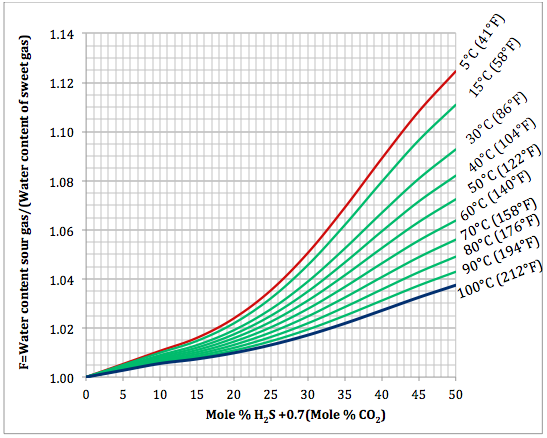Figure 1. Variation of sourness factor, F, with H2S equivalent concentration and temperature at 140 kPaa (20 psia)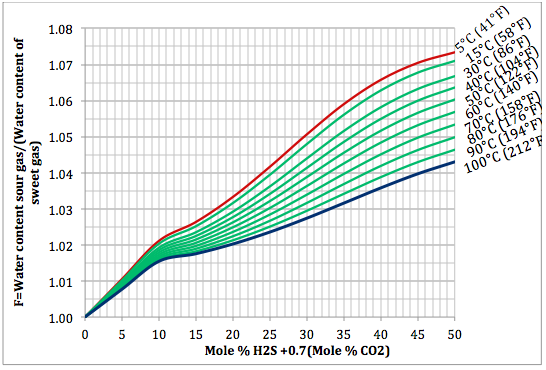Figure 2. Variation of sourness factor, F, with H2S equivalent concentration and temperature at 1400 kPaa (203 psia)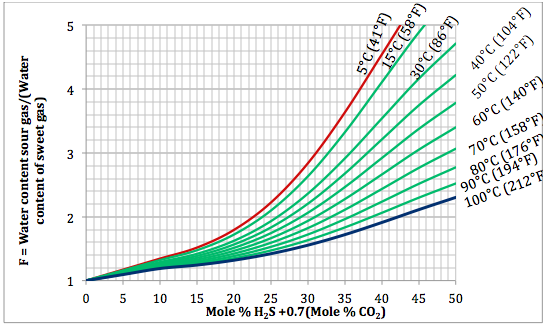Figure 3. Variation of sourness factor, F, with H2S equivalent concentration and temperature at 21000 kPaa (3045 psia)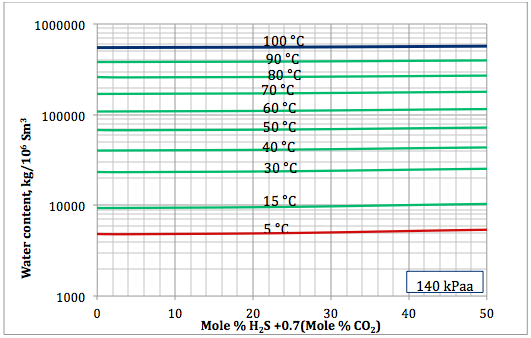Figure 4a. Sour gas water content as a function of H2S equivalent and temperature at 140 kPaa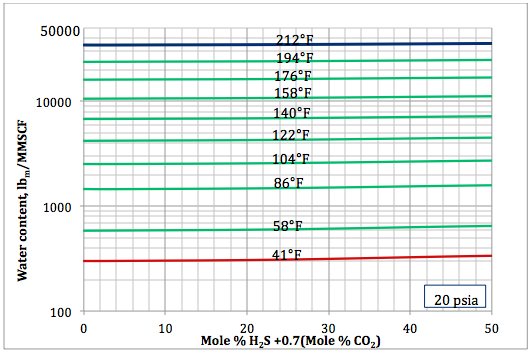Figure 4b. Sour gas water content as a function of H2S equivalent and temperature at 20 psia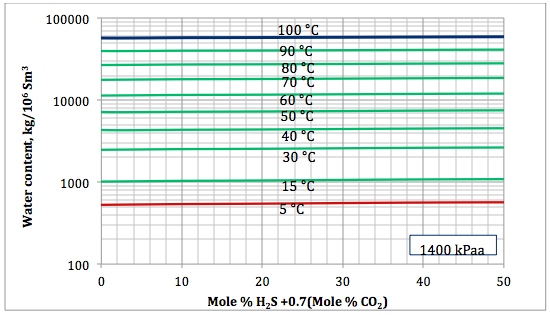Figure 5a. Sour gas water content as a function of H2S equivalent and temperature at 1400 kPaa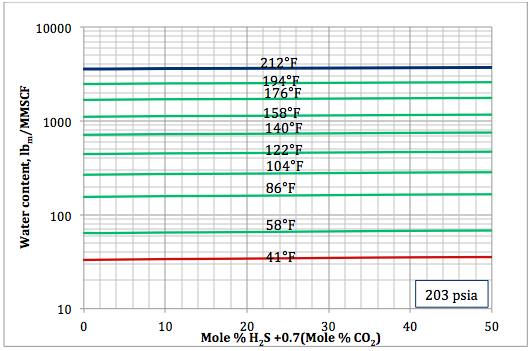Figure 5b. Sour gas water content as a function of H2S equivalent and temperature at 203 psia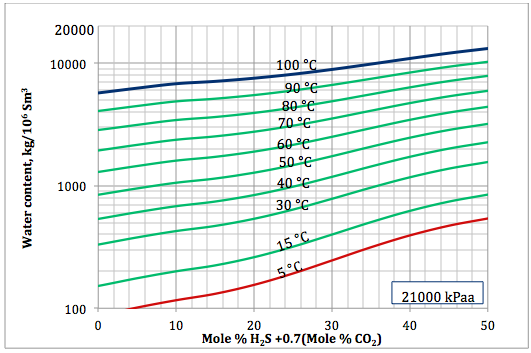Figure 6a. Sour gas water content as a function of H2S equivalent and temperature at 21000 kPaa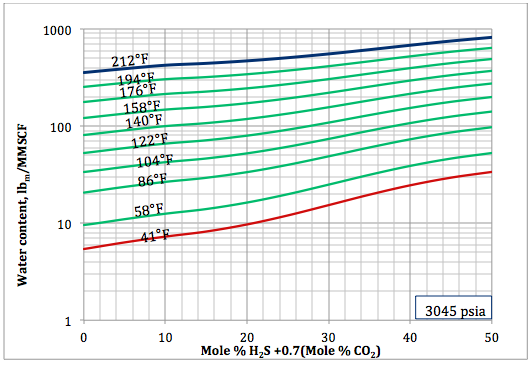Figure 6b. Sour gas water content as a function of H2S equivalent and temperature at 3045 psia

Model Evaluation

To evaluate the accuracy of the proposed correlations, the water content of several sour gas mixtures were predicted and compared against the experimental data and other shortcut and rigorous methods. The compositions of 12 sour gas mixtures for two isotherms and at pressure of 1380 kPaa (200 psia) along with the corresponding measured water contents from GPSA RR 174  studied in this TOTM are presented in Table 1. The predicted water contents by the proposed correlations are compared with these experimental data and the results obtained from Maddox et al.  and ProMax . The percent deviations between predicted results and the experimental values are presented in Table 1.

The calculated water content results by these three methods are also plotted against the experimental data in Figures 7 and 8 for the two isotherms of 48. 9 and 93.3°C (120 and 200°F), respectively. Figure 7 indicates that even though there are deviations between predicted values and the experimental data, the results of the three methods are in close agreement with each other. Figure 8 indicates better agreement between the predicted values and the experimental data but the agreement among the predicted values are not as close as in Figure 7.

Table 1. Comparison of prediction water content by the proposed correlations (W-W), Maddox et al. and ProMax with the experimental values  of several sour gas mixtures at 1380 kPaa (200 psia)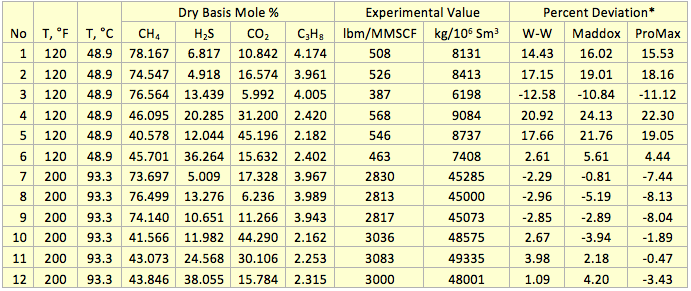* Percent deviation = 100(Experimental Value –Calculate Value)/(Experimental Value)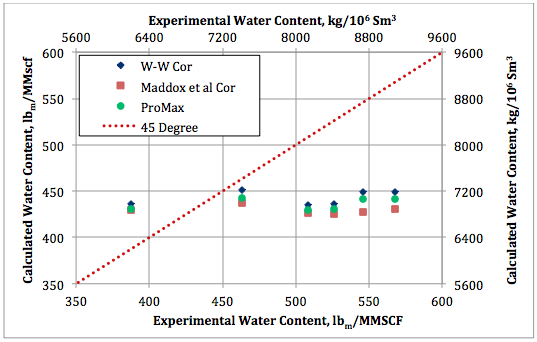Figure 7. Calculated water content by Wichert and Wichert, Maddox et al., and ProMax against experimental data at 48. 9°C (120°F) and 1380 kPaa (200 psia).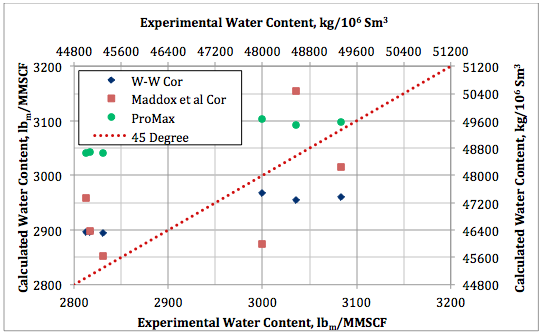Figure 8. Calculated water content by Wichert and Wichert, Maddox et al., and ProMax against experimental data at 93.3°C (200°F) and 1380 kPaa (200 psia).

Conclusions

The following conclusions can be made based on this case study:

1. Based on the Wichert and Wichert water content chart for sour gases a set of correlations (Equations 5 through 12) for spreadsheet calculation was developed and presented.
2. The proposed correlations are valid for pressures up to 24.14 MPa (3500 psia), temperatures up to 175°C (350°F) and H2S equivalent concentration of up to 50 mole %.
3. Based on the developed correlations, a set of easy-to-use charts (Figures 4 through 6) were prepared and presented which can be used to predict the water content of sour gas mixtures directly. Contrary to the original Wichert and Wichert chart, there is no need to look up water content of sweet gas.

To learn more about similar cases and how to minimize operational problems, we suggest attending our G4 (Gas Conditioning and Processing), G5 (Gas Conditioning and Processing – Special), G6 (Gas Treating and Sulfur Recovery) and PF81 (CO2 Surface Facilities), courses.

PetroSkills offers consulting expertise on this subject and many others. For more information about these services, visit our website at http://petroskills.com/consulting, or email us at consulting@PetroSkills.com.

By Dr. Mahmood Moshfeghian

Reference:

1. Campbell, J.M., “Gas conditioning and Processing, Vol. 1: The Basic Principles”, 9th Edition, 2nd Printing, Editors Hubbard, R. and Snow–McGregor, K., Campbell Petroleum Series, Norman, Oklahoma, 2014.
2. GPSA Engineering Data Book, Section 20, Volume 2, 13th Edition, Gas Processors and Suppliers Association, Tulsa, Oklahoma, 2012.
3. Maddox, R.N., L.L. Lilly, M. Moshfeghian, and E. Elizondo, “Estimating Water Content of Sour Natural Gas Mixtures”, Laurence Reid Gas Conditioning Conference, Norman, OK, Mar., 1988.
4. Wichert, G. C. and E. Wichert, “Chart Estimates Water Content of Sour Natural Gas,” Oil & Gas J., p. 61, Mar. 29, 1993.
5. Wagner, W. and Pruss, A.,  J. Phys. Chem. Reference Data, 22, 783–787, 1993.
6. Bukacek, R.F., “Equilibrium Moisture Content of Natural Gases” Research Bulletin IGT, Chicago, vol. 8, 198-200,       1959.
7. Ng, H.-J., C.-J. Chen, and H. Schroeder, “Water Content of Natural Gas Systems Containing Acid Gas”, Research Report RR-174, Gas Processors Association, Tulsa, OK, 2001.
8. ProMax 3.2, Bryan Research and Engineering, Inc., Bryan, Texas, 2014.